Courses

# Hydraulic Turbine, Buoyancy & Flotation - MCQ Test 2

## 30 Questions MCQ Test RRB JE for Mechanical Engineering | Hydraulic Turbine, Buoyancy & Flotation - MCQ Test 2

Description
This mock test of Hydraulic Turbine, Buoyancy & Flotation - MCQ Test 2 for Mechanical Engineering helps you for every Mechanical Engineering entrance exam. This contains 30 Multiple Choice Questions for Mechanical Engineering Hydraulic Turbine, Buoyancy & Flotation - MCQ Test 2 (mcq) to study with solutions a complete question bank. The solved questions answers in this Hydraulic Turbine, Buoyancy & Flotation - MCQ Test 2 quiz give you a good mix of easy questions and tough questions. Mechanical Engineering students definitely take this Hydraulic Turbine, Buoyancy & Flotation - MCQ Test 2 exercise for a better result in the exam. You can find other Hydraulic Turbine, Buoyancy & Flotation - MCQ Test 2 extra questions, long questions & short questions for Mechanical Engineering on EduRev as well by searching above.
QUESTION: 1

### The specific speed of an impulse hydraulic turbine will be greater than the specific speed of a reaction type hydraulic turbine.

Solution:

Ans. (b) Specific speed of impulse hydraulic turbine 10 – 35 rpm Specific speed of a reaction hydraulic turbine 300 – 1000 rpm

QUESTION: 2

### Specific speed of a Kaplan turbine ranges between

Solution:

Specific speed of a Kaplan turbine is equal to 51 to 225. Specific speed is denoted by Ns. It is used to characterize speeds in turbo machinery. Some of the main examples of turbomachinery are turbines. Specific speed plays an important role in the turbine.

QUESTION: 3

### Match List-I (Phenomena) with List-II (Causes) and select the correct answer: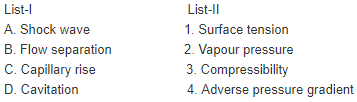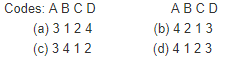Solution:

Ans. (c)

QUESTION: 4

Assertion (A): In turbo machines, stalling is a local phenomenon while surging affects the whole machine.
Reason (R): Stalling occurs when flow breaks away from the blades while surging causes complete breakdown of the flow.

Solution:

Ans. (a) Both A and R are true and R provides satisfactory explanation for A.

QUESTION: 5

The gross head on a turbine is 300 m. The length of penstock supplying water from reservoir to the turbine is 400 m. The diameter of the penstock is 1 m and velocity of water through penstock is 5 m/s. If coefficient of friction is 0.0098, the net head on the turbine would benearly

Solution:

Ans. (b)

QUESTION: 6

Match List-I (Flow parameter) with List-II (Type of turbine) and select the correct answer: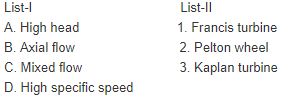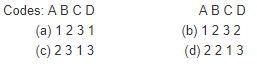Solution:

Ans. (c)

QUESTION: 7

Consider the following types of water turbines:
1. Bulb
2. Francis
3. Kaplan
4. Pelton
The correct sequence of order in which the operating head decreases while developing the same power is

Solution:

Ans. (a)

QUESTION: 8

Consider the following energies associated with a Pelton turbine:
1. Mechanical energy
2. Kinetic energy
3. Potential energy
The correct sequence of energy conversion starting from the entry offluid is:

Solution:

Ans. (c)

QUESTION: 9

Which one of the following statements regarding an impulse turbine iscorrect?

Solution:

Ans. (b)

QUESTION: 10

The gross head available to a hydraulic power plant is 100 m. The utilized head in the runner of the hydraulic turbine is 72 m. If the'hydraulic efficiency of the turbine is 90%, the pipe friction head is estimated to be:

Solution:

Ans. (a)

QUESTION: 11

As water flows through the runner of a reaction turbine, pressure acting on it would vary from:

Solution:

Ans. (a) Pressure of water in reaction turbine runner varies from more than atmospheric to vacuum. At runner inlet the pressure is more than atmospheric pressure and at runner outlet pressure is less than atmospheric (vacuum).

QUESTION: 12

Bodies in flotation to be in stable equilibrium, the necessary and sufficient condition is that the centre of gravity is located below the………………..

Solution:

Ans. (a)

QUESTION: 13

Assertion (A): The buoyant force for a floating body passesthrough the centroid of the displaced volume.
Reason (R): The force of buoyancy is a vertical force & equal tothe weight of fluid displaced.

Solution:

Ans. (a) When a solid body is either wholly or partially immersed in a fluid, the hydrostatic lift due to net vertical component of the hydrostatic pressure forces experienced by the body is called the buoyant force. The buoyant force on a submerged or floating body is equal to the weight of liquid displaced by the body and acts vertically upward through the centroid of displaced volume known as centre of buoyancy.
The x coordinate of the center of the buoyancy is obtained as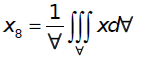Which is the centroid of the displaced volume. It is due to the buoyant force is equals to the weight of liquid displaced by the submerged body of volume and the force of buoyancy is a vertical force.

QUESTION: 14

What is buoyant force?

Solution:

Ans. (d) When a body is either wholly or partially immersed in a fluid, a lift is generated due to the net vertical component of hydrostatic pressure forces experienced by the body. This lift is called the buoyant force and the phenomenon is called buoyancy.

QUESTION: 15

Resultant pressure of the liquid in case of an immersed body acts through which one of the following?

Solution:

Ans. (b) For submerged body it acts through centre of buoyancy.

QUESTION: 16

Which of the following statement is true?

Solution:

Ans. (d) Here (a) is wrong: For an ideal fluid μ= 0, ρ = constant, K = const.
(b) is wrong: A floating body is in stable, unstable or neutral equilibrium according to as the metacentric height positive or negative or zero respectively. (c) is wrong: The exact analysis of viscous flow problems can be made by Navier stroke equations. Euler's equation is valid for nonviscous fluid.

QUESTION: 17

For floating bodies, how is the metacentric radius defined?

Solution:

Ans. (a) Metacentric Radius or Metacentric Height is the distance between Centre of Gravity and the Metacentre.

QUESTION: 18

Consider the following statements:
The metacentric height of a floating body depends
1. Directly on the shape of its water-line area.
2. On the volume of liquid displaced by the body.
3. On the distance between the metacentre and the centre ofgravity.
4. On the second moment of water-line area.
Of these statements correct are:

Solution:

Ans. (b) The metacentric height of a floating body depends on (2) and (3), i.e. volume of liquid displaced by the body and on the distance between the metacentre and the centre of gravity.

QUESTION: 19

Stability of a freely floating object is assured if its centre of

Solution:

Ans. (c)

QUESTION: 20

A cylindrical piece of cork weighting 'W' floats with its axis in horizontal position in a liquid of relative density 4. By anchoring the bottom, the cork piece is made to float at neutral equilibrium position with its axis vertical. The vertically downward force exerted by anchoring would be:

Solution:

Ans. (d) Due to own weight of cylinder, it will float upto 1/4th of its height in liquid of relative density of 4. To make it float in neutral equilibrium, centre of gravity and centre of buoyancy must coincide, i.e. cylinder upto full height must get immersed.
For free floating: Weight (W) = Buoyancy force (i.e. weight of liquid equal to 1/4th volume cork) The vertically downward force exerted by anchoring would be weight of
liquid equal to 3/4th volume cork = 3W.

QUESTION: 21

A large metacentric height in a vessel

Solution:

Ans. (d) Large metacentric height improves stability and decreases periodic time of oscillation.

QUESTION: 22

If a piece of metal having a specific gravity of 13.6 is placed inmercury of specific gravity 13.6, then

Solution:

Ans. (d)

QUESTION: 23

What are the forces that influence the problem of fluid static?

Solution:

Ans. (b) Gravity and pressure forces influence the problem of Fluid statics.

QUESTION: 24

A bucket of water hangs with a spring balance. if an iron piece issuspended into water from another support without touching the sides of the bucket, the spring balance will show

Solution:

Ans. (c)

QUESTION: 25

A metallic piece weighs 80 N air and 60 N in water. The relativedensity of the metallic piece is about

Solution:

Ans. (c)

QUESTION: 26

Which of the following advantages is/are possessed by a Kaplan turbine over a Francis turbine?
1. Low frictional losses.
2. Part load efficiency is considerably high
3. More compact and smaller in size.
Select the correct answer using the codes given below

Solution:

Ans. (d)

QUESTION: 27

Assertion (A): A Kaplan turbine is an axial flow reaction turbine withits vanes fixed to the hub.
Reason (R): Water flows parallel to the axis of rotation of the turbineand a part of the pressure energy gets converted to kinetic energyduring its flow through the vanes.

Solution:

Ans. (a)

QUESTION: 28

The use of a draft tube in a reaction type water turbine helps to:

Solution:

Ans. (c)

QUESTION: 29

Which of the following water turbines does not require a draft tube?

Solution:

Ans. (b)

QUESTION: 30

Which one of the following forms of draft tube will NOT improve the hydraulic efficiency of the turbine?

Solution:

Ans. (a)